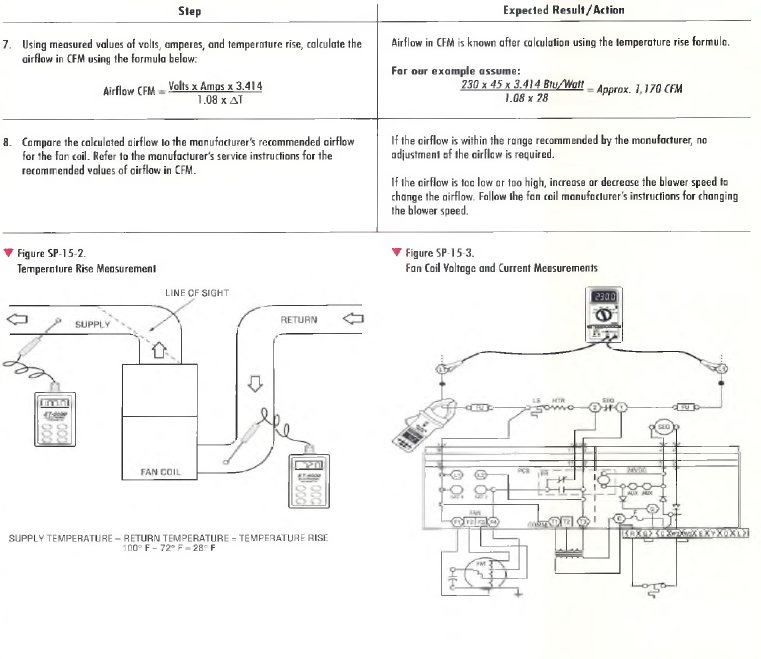﻿ AIRFLOW MEASUREMENT IN A FAN COIL WITH ELECTRIC HEAT | HVAC Troubleshooting

## AIRFLOW MEASUREMENT IN A FAN COIL WITH ELECTRIC HEAT

StepExpected Result/Action
1. Drill a hole in the return air duct near the fan coil as shown in Figure
SP-15-2. Drill a second hole in the supply air duct out of the line of sight of
Holes drilled in the supply and return air ducts in preparation for measurement.
2. Turn on power to the fan coil and set the thermostat to call for heat. Allow it
to run about ten minutes to stabilize the temperatures. Set the thermostat
high enough to cause the fan coil to run continuously during the measurement.
Fan coil on and temperatures stabilized.
3. Using the electronic thermometer, measure and record the return and supply
air temperatures. Make sure the thermometer readings have stabilized for
the measurement.
Supply and return air temperatures measured and recorded.
For our example assume:
Supply temperature = 1 0 0 ° F
Return temperature = 7 2 ° F
4. Using the measured values of supply and return temperatures, calculate the
temperature rise using the formula below:
Supply Temperature - Return Temperature = Temperature Rise
Temperature rise is calculated.
For our example assume:
100° F - 7 2 ° F = 2 8 ° F
5. Set up VOM/DMM to measure AC volts. Select a range high enough to
measure the fan coil input voltage. At the unit disconnect box or unit
terminal board, measure and record the input voltage as shown in
Figure SP-15-3.
Input voltage measured and recorded.
For our example assume:
The measured input voltage is 2 3 0 volts.
6. Set up the clamp-on ammeter to measure current on the highest range scale.
Measure and record the total current of the air handler as shown in Figure
SP-15-3.
Input current measured and recorded.
For our example assume:
The measured current is 4 5 amperes.Purpose – This procedure describes how to calculate the quantity of airflow in cubic feet per minute (CFM) being delivered by a fan coil containing electric heating elements. Having the correct quantity of airflow is important in order to maintain heating comfort in the conditioned space and for efficient and safe equipment operation. Airflow measurements are normally made when a fan coil is initially installed and when troubleshooting.

Calculating Airflow
The volume of airflow in a fan coil with electric heat can be calculated using measured values for the amount of temperature rise across the heating elements, along with the input voltage and the total (including blower motor) current flow through the air handler. Temperature rise is the difference between the return air temperature entering and the supply air temperature leaving the fan coil. While the measurements are being taken, the system must run continuously. The method for calculating airflow, including a typical example, is provided in the detailed procedure at the end of this section and briefly outlined here.

Before making any measurements, check that the air filter is clean, all supply and return registers are open and unrestricted, and that any zone dampers are fully open. Also, let the system run long enough to make sure the system temperatures have stabilized.

After the system is stabilized, the temperatures entering and leaving the unit are measured with an accurate electronic thermometer (Figure SP-15-1). N e x t, the temperature rise is calculated by subtracting the return air temperature from the supply air temperature. When measuring the discharge or supply air, the thermometer must be out of the line o f sight of the heater elements to prevent radiant heat from affecting the reading.

If the quantity of airflow in CFM is within the range specified by the equipment manufacturer, no adjustment of the airflow is required. If the flow is too low, the blower speed should be increased to increase the airflow. If it is too high, the blower speed should be decreased to decrease the airflow.